# Reflection of Light

## Types of Mirrors - Plain Mirror and Spherical Mirror

Reflection of light

Reflection is one of the distinctive properties of light. It is the reflection of light, which enables us to see anything
Reflection: The rebound of rays of light from an elegant and glossy surface is called reflection or reflection of light. It is related to a football bouncing back after colliding with a wall or any hard surface.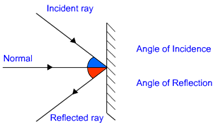Fig: Reflection of Light

Laws of Reflection of light:

• • The angle of reflection and incidence are equal.

• • The incident ray reflected ray and normal to the point of reflection lies in the same plane.

• The angle of incidence is denoted by 'i' and angle of reflection is denote by 'r'.  The rule of reflection is valid to all type of reflecting surface.

Mirror and Reflection of Light:

The mirror is a shiny polished object (glass) which reflects most of the rays of light falling upon it One side of the mirror is cleaned with an appropriate material to make the opposite side reflective.

Types of Image formed by mirrors:

Real Image:
Picture which is framed before the mirror and it very well may be acquired on a screen is called a genuine picture.
Virtual Image: Picture which is framed before the mirror and it very well may be acquired on a screen is called a genuine picture.

Types of Mirror:

Plain Mirror:
A mirror having a level surface is known as a plane mirror.

Formation of an image in the plane mirror: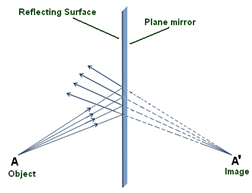Fig: Image formation in a plain mirror

• • A plane mirror dependably frames a virtual and erect picture.

• • The separation of the picture and that of an item is equivalent from the mirror.

• • The picture shaped by a plane mirror is horizontally modified.

• Spherical Mirror

Mirrors having a curved reflecting surface are called round mirrors. A plane mirror constantly outlines a virtual and erect picture.

• • The partition of the image and that of a thing is equal from the mirror.

• • The picture formed by a plane mirror is evenly adjusted. A round mirror is a piece of a circle.

• Kinds of Spherical Mirror:

Concave Mirror: Spherical mirror with a reflecting surface bent inwards is known as a concave mirror.
Convex Mirror: Spherical mirror with a reflecting surface bent outwards is known as a convex mirror.

Important terms in the case of a spherical mirror: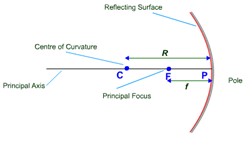Fig: Concave Mirror

Pole: The focal point of reflecting surface of a round mirror is known as Pole. Pole lies on the outside of the round mirror. It is commonly known by 'P'.

Centre of Curvature: The focal point of a circle; of which the reflecting surface of a round mirror is a section; is known as the center of curvature of the spherical mirror. Centre of curvature is not an element of a spherical mirror. Centre of curvature is indicating by letter ‘C’.

On account of the concave mirror center of curvature lies before the reflecting surface. Then again, Center of curvature lies behind the reflecting surface on account of a convex mirror.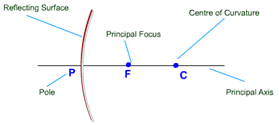Fig: Convex Mirror
The radius of Curvature: The radius of the circle; of which the reflecting surface of a circular mirror is a section; is known as the Radius of Curvature of the round mirror. The range of curvature of a spherical mirror is signified by letter ‘R’.  Like the center of curvature, the radius of shape lies before the concave mirror and lies behind the convex mirror and isn't a piece of the mirror as it lies outside the mirror.

Aperture: The diameter across from the reflecting surface of a round mirror is called aperture.
Principal Axis: Imaginary line going through the center of curvature and pole of a round mirror are known as the Principal Axis.
Focus or Principal Focus:  The point on a principal axis which parallel rays; originating from infinity; meet after reflection is known as the Focus or Principal Focus of the round mirror. It is signified by letter “F”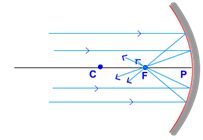Fig: Converging Mirror
In a concave mirror, similar rays; coming from infinity; converge after reflection at the front of the mirror. Thus, the center point lies in front of a concave mirror.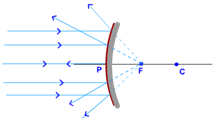Fig: Diverging Mirror

In a convex mirror, equivalent rays; coming from infinity; emerge to be diverging from at the rear mirror. Thus, the focal point lays at the rear of the convex mirror.

Focal length: The space from pole to focus is called focal length. Focal length is indicating by the letter ‘f'. Focal length is equivalent to half of the radius of curvature.
Or, f=R2f=R2
Or, R=2f

Reflection from a spherical mirror:

Reflection of Rays parallel to Principal Axis:

In the case of a concave mirror: A Ray equivalent to the principal axis passes during the principal focus after reflection from a concave mirror.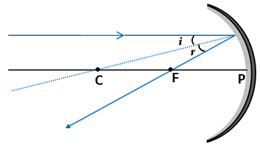Fig: Rays parallel to the principal axis

In the same way, all equivalent rays to the principal axis pass through the principal focus after reflection from a concave mirror. Because of a concave mirror converge the similar rays after reflection, thus a concave mirror is also known as a converging mirror.

In the case of a convex mirror: A  ray parallel to the principal axis appears to move away from the principal focus after reflecting from the surface of a convex mirror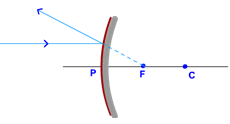Fig: Rays parallel to the principal axis

Likewise, all rays similar to the principal axis of a convex mirror appear to move away or coming from principal focus after reflection from a convex mirror. Since a convex mirror diverges the similar rays after reflection, thus it is also known as a diverging mirror.

Reflection of a ray passing through the Principal Focus:

In the case of the concave mirror:
Ray going through the principal focus goes parallel to the principal axis after the appearance on account of the concave mirror.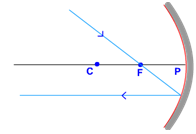Fig: Ray passing through the principal focus

In the case of the convex mirror: A ray guided towards principal focus goes parallel to the principal axis after reflecting from the outside of a convex mirror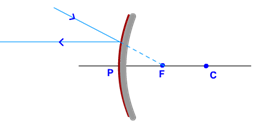Fig: Ray through principal focus
Ray passing through the Centre of curvature:

In the case of the concave mirror: Beam going through the center of curvature returns at a similar way subsequent to reflecting from the outside of an inward mirror.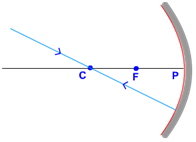Fig: Ray passing through C<
In the case of the convex mirror: Beam seems to go through or coordinated towards the center of curvature parallel to the principal axis after reflecting from the surface of a convex mirror
.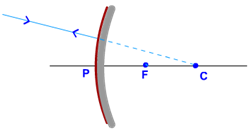Fig: Ray passing through C
Ray incident obliquely to the principal axis: Ray on a slope to the principal axis goes indirectly after reflecting from the pole of both concave and convex mirror and in the same direction.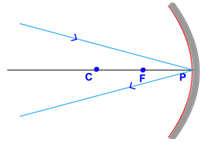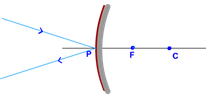Fig: Ray passing obliquely to the principal axis
Image Formation by Concave Mirror

The arrangement of the image relies upon the place of the object. In the case of the concave mirror, there are six possibilities on which position of the object is placed.

• a. Object at infinity

• b. The object between infinity and the center of curvature (C)

• c. An object at the center of curvature (C)

• d. The object between the center of curvature (C) and Principal focus (F)

• e. An object at Principal Focus (F)

• f. Object among Principal Focus (F) and Pole (P)

• An object at infinity:

Since parallel rays coming from the object converge at the principal focus, F of a concave mirror; after reflection. Hence, when the object is at infinity the image will form at F.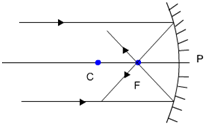Fig: Object at Infinity
Properties of the image:
• • Point sized

• • Highly diminished

• • Real and inverted

• The object between infinity and Centre of Curvature:

When the object is positioned between infinity and center of curvature of a concave mirror the image is created between the center of curvature (C) and focus (F).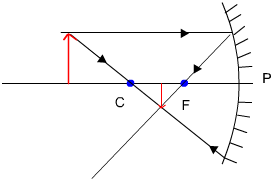Fig: Object Between Infinity and C

Properties of the image:

• • Diminished compared to the object

• • Real and inverted

• An object at the Centre of Curvature (C):

When the object is located at the center of curvature (C) of a concave mirror, a real and inverted image is created at a similar position.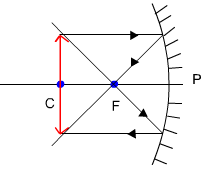Fig: Object at C

Properties of the image:

• • Same size as the object

• • Real and inverted

• Object among Centre of curvature (C) and Principal Focus (F):

When the object is to be found between the center of curvature and the principal focus of a concave mirror, a real image is created beyond the center of curvature (C).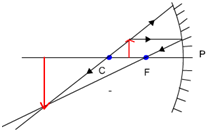Fig: Object between C and F

Properties of the image:

• • Larger than object

• • Real and inverted

• An object at Principal Focus (F):

When the point is located at the principal focus (F) of a concave mirror, a highly inflated image is created at infinity.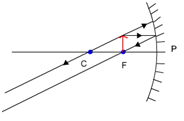Fig: Object at F

Properties of the image:

• • Highly enlarged

• • Real and inverted

• The object between Principal Focus (F) and Pole (P):

When the point is located between principal focus and pole of a concave mirror, an inflamed, virtual and erect image is created behind the mirror.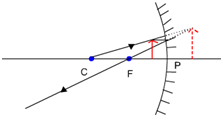Fig: Object between F and P

Properties of the image:

• • Enlarged

• • Virtual and erect

• Image Formation by Convex Mirror

Two possibilities only are possible of the position of the object in the case of a convex mirror, i.e. the object at infinity and object among infinity and pole of a convex mirror.

An object at infinity: When the object is at the infinity, a point-sized image is formed at principal focus behind the convex mirror.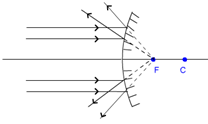Fig: Object at infinity

Properties of image: Image is extremely diminished, virtual and erect.

The object between infinity and pole: When the object is among infinity and pole of a convex mirror, a diminished, virtual and erect image is created among pole and focus behind the mirror.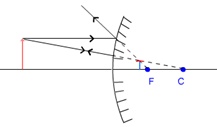Fig: Object between infinity and P

Characteristics of object: object is diminished, virtual and erect.